While not all of your employees need to be Excel experts, you don't want them to be totally baffled when they open up a spreadsheet.

Wouldn't it be valuable if all of your team members could at least interpret any simple excel formulas they encounter?

In a previous post I explained how to build a simple formula and view a formula. Today, I want to cover how to build and interpret a formula using more than one operator (+, -, *, /). This can be challenging especially if you are not familiar with the order of operations, which dictates how calculations are performed.

By the way, I'm a certified Excel instructor. If you like this post, please consider taking a class with me!

## Build a formula using more than one operator

In the following example, I am looking to find the total price due in cell D2. To make things a little more challenging there is a \$20 discount and 7% tax that needs to be applied to the formula.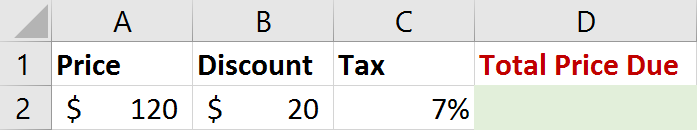Steps to build the basic formula:

1. Click in the cell D2 and type in the = sign (all formulas begin with the = sign).
2. Select cell A2 (this is the first cell you need to add to the formula - you should notice 'marching ants' around the selected cell).
3. Type the subtraction (-) sign on the keyboard, then click cell B2 (we need to subtract the \$20 from the \$120 before applying the 7% sales tax).
4. Type the multiplication (*) sign, then click cell C2.
Your formula should look like this =A2-B2*C2
The formula at this point will only return the sales tax amount. We are looking for the total price due after applying the discount and adding in the sales tax. This requires adding back in the purchase price minus the discount.
5. Type the addition (+) sign, then click cell A2.
6. Type the subtraction (-) sign, then click cell B2.
7. Confirm the formula by hitting the Enter key on the keyboard.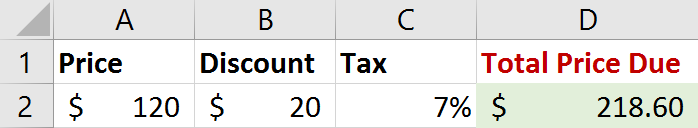## Whoops! There is a lesson to be learned here...

I was expecting an answer of \$107.00 not \$218.60. So, what went wrong? Let’s take a closer look at the formula and examine the order in which Excel did its calculation.

=A2-B2*C2+A2-B2## The Order of Operations

When calculating simple Excel formulas (or even complex formulas), Excel follows the order of operations which determines the order that calculations are performed in a formula.

Below is a table showing the order of precedence. It goes: parenthesis, exponents, multiple and division, then finally addition and subtraction.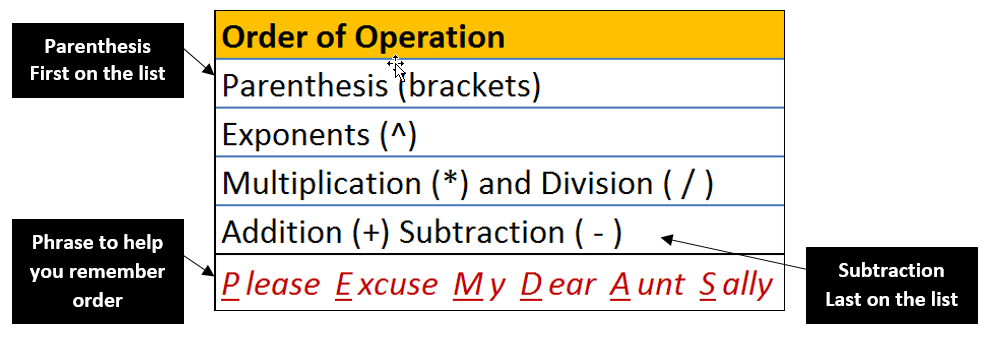You will notice that multiplication comes before subtraction. The problem in our example is that we need to subtract the discount first before multiplying the sales tax.

Since we need the subtraction calculated first we will need to wrap that part of the formula in parenthesis. Any part of a formula wrapped in parenthesis is calculated first.

The formula should look like this:

=(A2-B2)*C2+(A2-B2)

Here's a breakdown of how the order of operations will be applied to this updated simple Excel formula.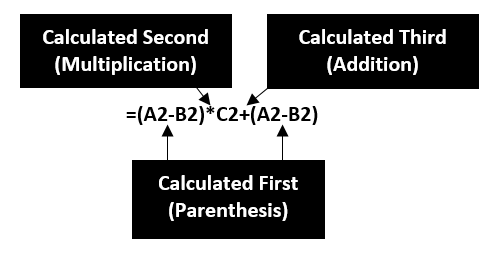This will give us the correct answer of \$107.00 due.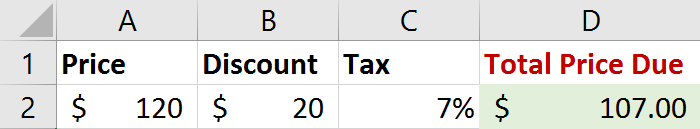## You might also like:Topics: Excel